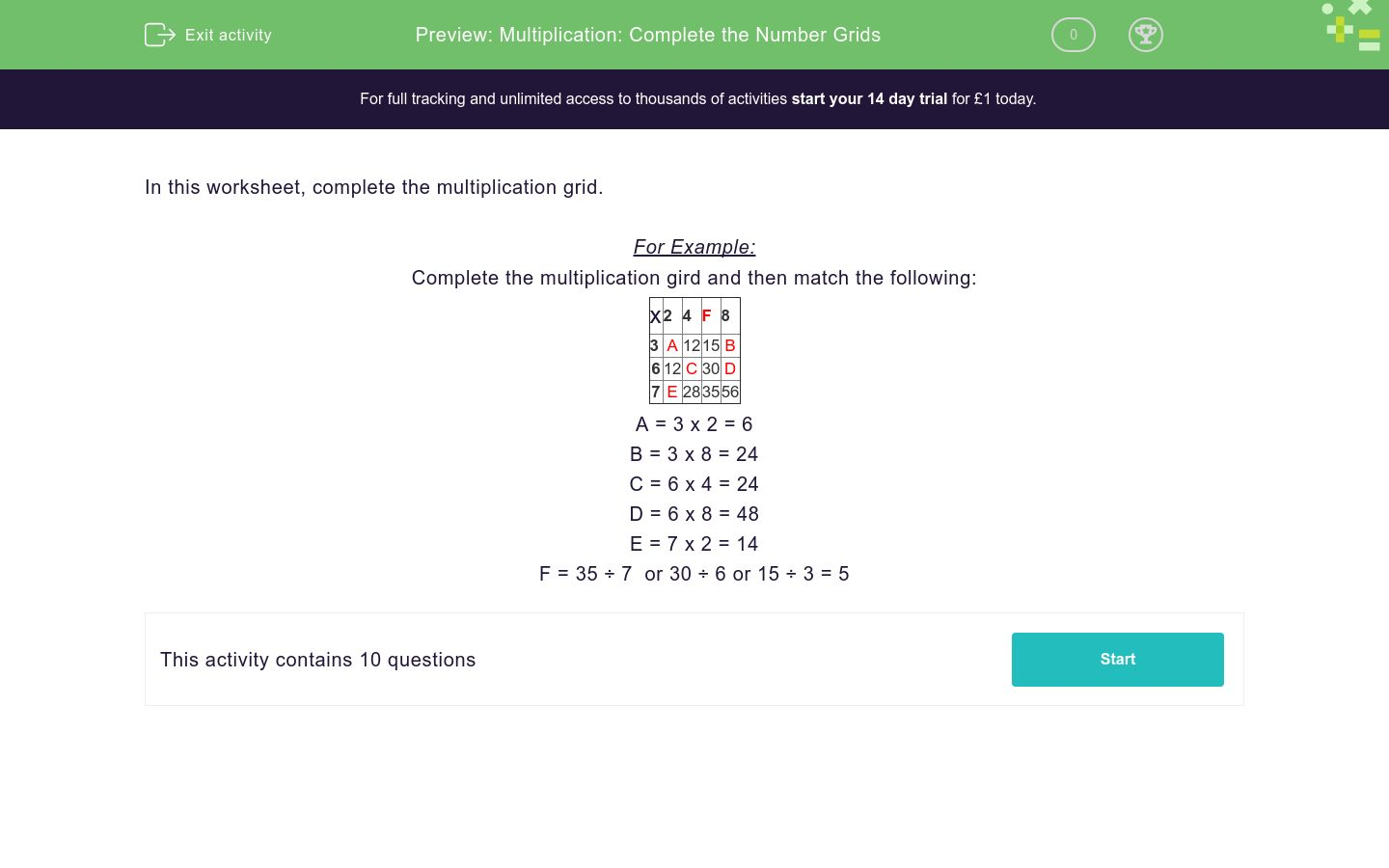# Multiplication: Complete the Number Grids

In this worksheet, students use both multiplication and division in order to complete a grid of numbers.Key stage:  KS 2

Curriculum topic:  Number: Addition, Subtraction, Multiplication and Division

Curriculum subtopic:  Carry Out Calculations Using Order of Operations

Difficulty level:### QUESTION 1 of 10

In this worksheet, we have to complete the multiplication grid.

For Example:

Complete the multiplication gird and then match the following:

x

2 4 F 8
3 A 12 15 B
6 12 C 30 D
7 E 28 35 56

A = 3 x 2 = 6

B = 3 x 8 = 24

C = 6 x 4 = 24

D = 6 x 8 = 48

E = 7 x 2 = 14

F = 35 ÷ 7  or 30 ÷ 6 or 15 ÷ 3 = 5

Complete the multiplication gird and then match the following:

x

2 4 5 8
3 A 12 15 B
6 12 C 30 D
7 E 28 F 56

## Column B

A
24
B
24
C
14
D
48
E
6
F
35

Complete the multiplication gird and then match the following:

x

A 3 5 8
4 8 12 B 32
6 12 C 30 D
9 E 27 45 F

## Column B

A
72
B
18
C
18
D
48
E
20
F
2

Complete the multiplication gird and then match the following:

x

7 A 5 3
2 14 8 15 B
6 12 C 30 D
E 56 32 F 24

## Column B

A
8
B
4
C
40
D
24
E
18
F
6

Complete the multiplication gird and then match the following:

x

5 6 7 A
3 15 12 15 B
5 12 C D 45
E 45 54 63 F

## Column B

A
9
B
27
C
81
D
30
E
35
F
9

Complete the multiplication gird and then match the following:

x

2 C 5 8
3 A 18 15 B
6 12 36 30 D
E 20 60 F 64

## Column B

D
50
F
6
A
48
C
24
E
6
B
10

Complete the multiplication gird and then match the following:

x

A 8 F 5
8 24 64 56 40
E 12 C 28 D
7 21 B 49 35

## Column B

D
7
F
56
C
4
E
20
B
3
A
32
• Question 1

Complete the multiplication gird and then match the following:

x

2 4 5 8
3 A 12 15 B
6 12 C 30 D
7 E 28 F 56

## Column B

A
6
B
24
C
24
D
48
E
14
F
35
EDDIE SAYS
Find the value of the letters using your times table facts.
• Question 2

Complete the multiplication gird and then match the following:

x

A 3 5 8
4 8 12 B 32
6 12 C 30 D
9 E 27 45 F

## Column B

A
2
B
20
C
18
D
48
E
18
F
72
EDDIE SAYS
Find the value of the letters using your times table facts. You will have to divide to find the value of A.
• Question 3

Complete the multiplication gird and then match the following:

x

7 A 5 3
2 14 8 15 B
6 12 C 30 D
E 56 32 F 24

## Column B

A
4
B
6
C
24
D
18
E
8
F
40
EDDIE SAYS
Find the value of the letters using your times table facts.
• Question 4

Complete the multiplication gird and then match the following:

x

5 6 7 A
3 15 12 15 B
5 12 C D 45
E 45 54 63 F

## Column B

A
9
B
27
C
30
D
35
E
9
F
81
EDDIE SAYS
Find the value of the letters using your times table facts.
• Question 5

Complete the multiplication gird and then match the following:

x

2 C 5 8
3 A 18 15 B
6 12 36 30 D
E 20 60 F 64

## Column B

D
48
F
50
A
6
C
6
E
10
B
24
EDDIE SAYS
Find the value of the letters using your times table facts.
• Question 6

Complete the multiplication gird and then match the following:

x

A 8 F 5
8 24 64 56 40
E 12 C 28 D
7 21 B 49 35

## Column B

D
20
F
7
C
32
E
4
B
56
A
3
EDDIE SAYS
Find the value of the letters using your times table facts.
---- OR ----

Sign up for a £1 trial so you can track and measure your child's progress on this activity.

### What is EdPlace?

We're your National Curriculum aligned online education content provider helping each child succeed in English, maths and science from year 1 to GCSE. With an EdPlace account you’ll be able to track and measure progress, helping each child achieve their best. We build confidence and attainment by personalising each child’s learning at a level that suits them.

Start your £1 trial